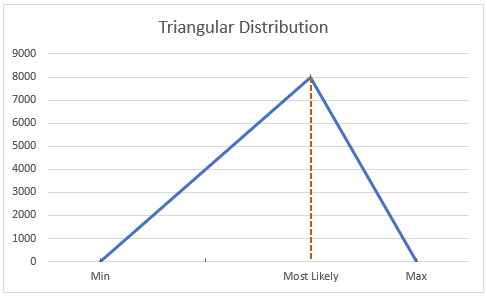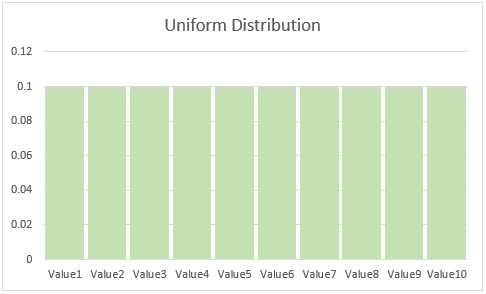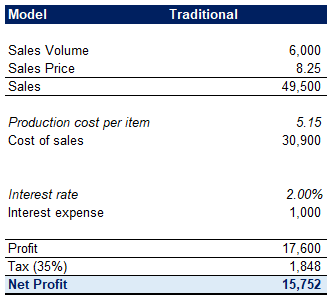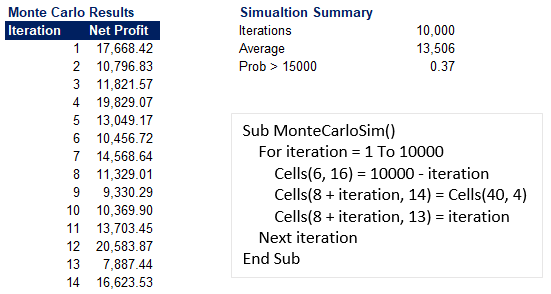## Monte Carlo Simulation in Financial Modeling

Whenever we are constructing a financial model, we rely heavily on assumptions. Some, if not all, of those assumptions, have the associated uncertainty and inherent risk. Not being able to predict the future makes it harder to solve and model the probability of different outcomes from our financial models.

In such situations, we can apply a Monte Carlo Simulation to analyze the effect of randomness introduced by such variables in our model.

The simulation works by performing repetitive calculations using random inputs for these assumptions and then averages out the most probable output of the model.

## What is the Monte Carlo Simulation

In the 1940’s Stanislaw Ulam, a Polish scientist, developed the method, to be later used in the Manhattan Project. The similar randomness to games like roulette is behind the technique receiving the name of the Monaco casino.It is a stochastic method, which means it uses random samples of input values, and it solves a statistical problem.

We can apply the Monte Carlo Simulation to almost any problem with probability. Therefore analysts use it widely in many fields like finance, statistics, manufacturing, engineering, R&D, biology, and others. The technique has many applications in finance, some of which are:

• Valuation of equity options;
• Portfolio valuation;
• Sensitivity analysis in financial modeling;
• Uncertain future cash flows calculation;
• Pricing stocks.

The Monte Carlo Simulation is a tool for risk assessment that aids us in evaluating the possible outcomes of a decision and quantify the impact of uncertain variables on our models. The method allows analysts to gauge the inherent risk in decision-making and quantitative analysis.

It is a very flexible technique, as we can vary the assumptions for as many variables as we want and end up with a range of probable outcomes for our forecasts.

A downside to the method that analysts often point out is the inability of the Monte Carlo Simulation to allow for any kind of financial crisis that may affect the inputs. It also cannot factor in the irrationality displayed by participants in the market we are analyzing.

## Probability Distributions

The Monte Carlo Simulation uses various probability distributions to calculate uncertain factors. By doing so multiple times, it produces a distribution of the possible output values of the model. We use probability distributions that appropriately match the different assumptions. That way, the variables can have different probabilities for different amounts.

Let’s look at some of the most common probability distributions we use in financial modeling.

### Normal DistributionThe normal distribution is also known as the ‘bell curve’ distribution. It is symmetrical and applies to natural phenomena like people’s weight or height. When we use this distribution for a variable in our financial model, we define a mean, which represents the value we expect to occur, and a standard deviation, to quantify the variation of the factor. When we apply this distribution, values closer to the mean are more likely to occur. In financial modeling, analysts would use it for inflation and interest rates, prices of fuel and electricity, and others.

### Triangular DistributionFor this distribution, we need to define a minimum, maximum, and most likely value. Similarly to the normal distribution, values closer to the most likely option will be more likely to occur. Analysts apply the triangular distribution to periodic sales performance, inventory levels, work hours, and others.

### Uniform DistributionWhen we have a variable, where all possible values have the same equal chance of occurring, we apply a uniform distribution. For this distribution, we only identify the minimum and maximum, and each value in between has the same probability. In financial modeling, assumptions with uniform distributions can be future production costs and selling prices for new products.

### Discrete Distribution

In this distribution, we define specific values and the chance of their outcome. An example can be the passing of new legislation for additional sales tax, for which we can describe:

• [30%] Passed – applicable for sales from 01/01/2020;
• [20%] Passed – applicable for sales from 01/07/2020;
• [50%] Rejected.

## Performing a Monte Carlo Simulation

In theory, it is quite easy to perform the Monte Carlo Simulation. The way the method works is by following these steps:

1. Assign a random value to the variable, for which we cannot calculate the probabilities;
2. Calculate the model with this random value for the assumption;
3. Record the result;
4. Change the random value for the variable;
5. Recalculate and re-iterate for hundreds, or even thousands of times;
6. Average out the result

During each iteration of the simulation, we select random values from the distribution population of each variable.

The result of the Monte Carlo Simulation is a probability distribution, or an array of sorts, of all possible outcomes from our model. It provides a more comprehensive outlook on what may happen and how likely it is to happen.

## Advantages to the Monte Carlo Method

The Monte Carlo Simulation has some distinct advantages over traditional analysis, relying on one set of assumptions.

• Results we derive have probabilities;
• A better sensitivity analysis that not only shows the result for a few changes in the selected assumptions but also which inputs have the most significant impact on outputs;
• We can easily present the data from Monte Carlo Simulations graphically, for when we have to communicate findings to stakeholders;
• Monte Carlo Simulations show us the exact combination of values for each variable that contributed to a specific outcome of the model. At the same time, with traditional scenario analysis, it’s often hard and time-consuming to prepare a whole set of values for all the different inputs.

## Monte Carlo Simulation in Excel

Let us illustrate the implementation of Monte Carlo Simulations in our financial models by applying the method to a simple Excel model for a project.

We have the following assumptions for our model, where we have also added the Probability distributions that they follow.Our goal is to reach a € 15,000 result in the first year, which will be enough to cover our fixed costs and remain profitable. For the sake of simplicity, we will only consider the above assumptions.

If we follow the traditional forecasting approach, we use our variables to arrive at the following model.In our calculation, we take the most likely sales volume value and the average production cost per item. For our interest expense, we use the mean (the expected value), and for our tax rate, we employ a weighted tax percentage.

In doing so, we arrive at a profit of above € 15,000. If we only consider this analysis, we would expect the venture to be profitable and go ahead with it.

Now, let’s add a Monte Carlo Simulation to our model.We have added the distributions for the uncertain variables and introduced a random element in each of them. Now, every time we press F9, the random values are recalculated, and this changes our model output. As you can see, it is currently showing us a worse result than our traditional approach. However, by hitting F9 and recalculating the sheet, we will sometimes get far better results.

The whole idea of a Monte Carlo Simulation is to re-iterate these calculations many times. Let us employ a simple macro that will do 10,000 iterations and record the result for each one.The random values in the simulation change every iteration the macro runs. It then copies the Net Profit to our list of outputs. We then employ simple formulas to calculate some summary metrics.

We can see that averaging the results out, and we get a result below the required € 15,000. Also, the probability of reaching our hurdle profitability is 37%. Implementing the Monte Carlo Simulation to our financial model shows us that the project is not a viable one.

It is hard to be sure if the iterations are enough, so let us look if there would be any significant changes to our findings if we simulate 100,000 iterations instead.As you can see, simulating ten times more iterations yields similar results.

## Conclusion

The Monte Carlo Simulation is a stochastic method to account for the inherent uncertainty in our financial models. It has the benefit of forcing all engaged parties to recognize this uncertainty and think about probabilities, rather than simple values.

When we perform a Monte Carlo Simulation, we describe the uncertain variables with the help of appropriate probability distributions. However, the technique is not widely used, as daily financial software like Excel doesn’t have it built-in. We either need to develop our in-house model or purchase specialized software, which is why many financial professionals don’t have the method in their toolbox.

Nonetheless, the simulation is a very flexible and robust modeling technique that can significantly improve our sensitivity analysis and overall financial modeling. In essence, the Monte Carlo Simulation allows us to transform the chance in choice.

## And Get a FREE Benchmark Analysis Template## FCCA, FMVA

Hi! I am a finance professional with 10+ years of experience in audit, controlling, reporting, financial analysis and modeling. I am excited to delve deep into specifics of various industries, where I can identify the best solutions for clients I work with.

In my spare time, I am into skiing, hiking and running. I am also active on Instagram and YouTube, where I try different ways to express my creative side.